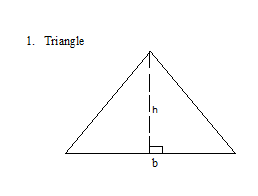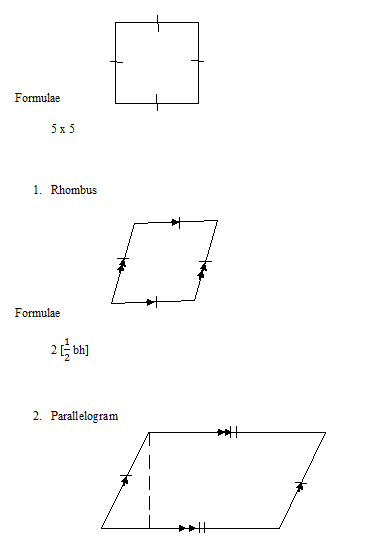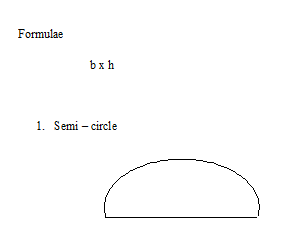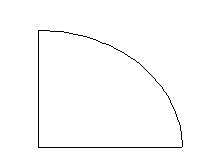# PHY1: AREA

##### This Unit is about the Area as calculated in Olevel Physics.

AREA

Area is the region under or within a boundary of an object. Area is measured in square units e.g. cm3, m2, mm2.

Where

1km2 =? m2

[1km]2 = [1000m]2

1km2 = 1000m x 1000m

1km2 = 1,000,000m2

And

1m2 =? cm2

[1m] 2 = [100cm]2

1m2 = 1000cm x 100cm

1m2 = 10,000cm2

FORMULAE FOR FINDING AREA OF OBJECTS

ObjectFormulae

1/2  x b x h

RectangleFormulae

Length x width

SquareFormulae

1/2 x r2Formulae

1/4 x r2

SURFACE AREA

It is the total area around the surface of an object. It is obtained by either adding up the area of the faces on using formulae.

EXAMPLESFormulae

2 x r2   = [r +h]xr2### ASSIGNMENT : AREA assignmentMARKS : 10  DURATION : 1 week, 3 days

SEE ALL
•YOU